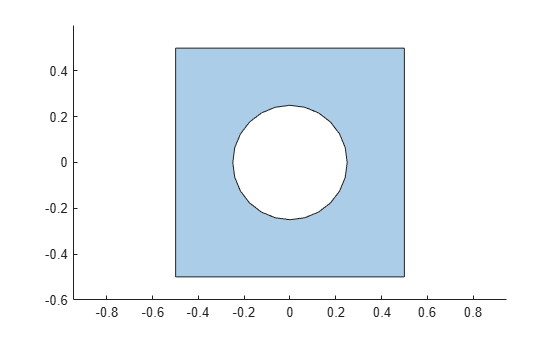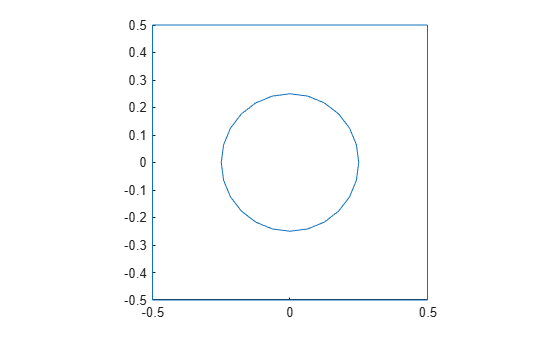# Geometry from `polyshape`

This example shows how to create a polygonal geometry using the MATLAB® `polyshape` function. Then use the triangulated representation of the geometry to create an `fegeometry` object.

Create and plot a `polyshape` object of a square with a hole.

```t = pi/12:pi/12:2*pi; pgon = polyshape({[-0.5 -0.5 0.5 0.5], 0.25*cos(t)}, ... {[0.5 -0.5 -0.5 0.5], 0.25*sin(t)})```
```pgon = polyshape with properties: Vertices: [29x2 double] NumRegions: 1 NumHoles: 1 ```
```plot(pgon) axis equal```Create a triangulation representation of this object.

`tr = triangulation(pgon);`

Create a geometry by using the triangulation data.

`gm = fegeometry(tr)`
```gm = fegeometry with properties: NumFaces: 1 NumEdges: 5 NumVertices: 5 NumCells: 0 Vertices: [5x3 double] Mesh: [1x1 FEMesh] ```

Plot the geometry.

`pdegplot(gm);`Because the triangulation data results in a low-quality linear mesh, generate a new finer mesh for further analysis.

```gm = generateMesh(gm); gm.Mesh```
```ans = FEMesh with properties: Nodes: [2x1244 double] Elements: [6x572 double] MaxElementSize: 0.0566 MinElementSize: 0.0283 MeshGradation: 1.5000 GeometricOrder: 'quadratic' ```

Plot the mesh.

`pdemesh(gm);`